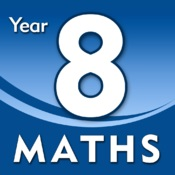Year 8Topics taught in Year 8 include :-

Number Properties & Calculations ... Multiplication, brackets in calculations, direct proportion, ratio notation, prime factor decomposition, multiply & divide negative integers, reducing ratio, £ and pence, square roots, equivalent ratios, HCF & LCM, ratio & fractional notation, unitary methods, squares cubes & roots, positive power, giga mega & kilo, numerator & denominator of fractions.

Fractions, Decimals, Percentages & Ratio ... Identification. mental calculation, decimal numbers, + & -, halving & doubling strategies, percentages & comparison, quantites & measurement, positive fractions, simplifying fractions, round & order decimals, multiply integrers & fractions by a fraction, mental strategies, fractional equivalents, division, squares of numbers, simplify ratio, calculate with mixed numbers, use inverse operation, lists, unitary method for an inverse operation, reciprocal of a number, recurring decimals, percentage change, compound interest & repeated percentage change.

Shapes, Measures in 2D & 3D ... Names & use of 3D shapes, 2D representations of 3D shapes, prism, faces, edges & vertices, nets of closed cubes & cuboids, formula for volume of cube & cuboid, geometric properties, area of triangles, parallelogrames & trapezia, calculate surface areas, using maths equip in construction, length, area & capacity, metric and imperial conversion, analysing shapeas informally & through cross-sections, plans & elevations, indentifying the hypotenuse, names & parts of a circle, solving volume problems, radius & diameter, Pythagorus' theorum, cylinders.

Statistics & Graphs ...  Frequency tables, bar graphs, data, pie charts, data-logging, modal class of a set if continuous data, scatter graphs, correlation, stem & leaf diagrams to find mode, median, mean & range, using complex two way tables, linear & non-linear graphs, conversion graphs, proportion, straight line graphs, rates of change,

Expressions & Equations ... Distributive law with brackets & numbers, expression, equation and the meaning of the key vocabulary 'term', algebra, linear terms, formulae, powers, distributive law, numerical common factors, index notation, brackets & powers, index law & powers of variables.

Angles & Construction ... Reflex angles, using a protractor, calulation of angles, using a ruler in angles, investigating using Pythagoris theorum, classifying quadilaterals, sum of angles, geometric problems and properties, reasoning, equations, construction - midpoints and perpendicular bisector of a line segment, RHS, locus equidistant points, surface of shape,

Probability ... probablilty scale, finding and justifying probablilty, identification, estimation, relative frequency, sum of outcomes, tree diagrams, calculation.

Straight Lines & Functions ... Finding midpoints, gradients, plotting graphs of linear functions, finding the inverse, straight line graphs, geometric sequences, features of graphs, parallel/perpendicular equation, contextual problem solving, piece-wise linear, exponential & reciprocal graphs.

Click in the link below to access a copy of the student booklet which is issued to all Maths students in Year 8 at the start of the academic year.